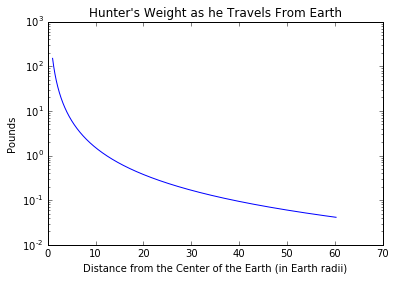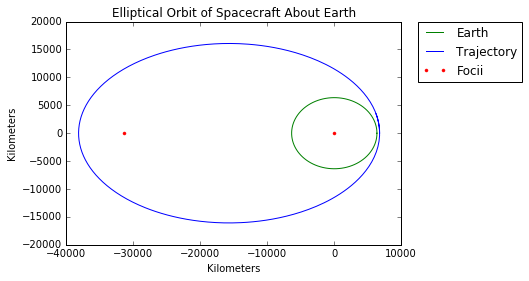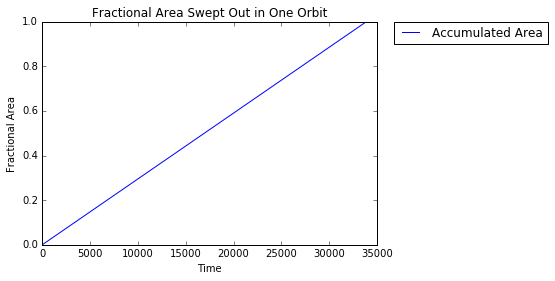# Astro 1102/1104, Section 1¶

In :
from IPython.display import Latex
import matplotlib.pyplot as plt
%matplotlib inline
import numpy
from matplotlib import animation, rc
from IPython.core.display import HTML
from IPython.display import HTML
from ipywidgets import *
from scipy.integrate import odeint


## General Information¶

#### My contact/office hours¶

NetID: vha3

Office Hours: Tuesdays, 10:00 - Noon (Space Sciences)

Attendance, participation, quizzes?

Homework will be posted to Blackboard on Mondays, and will be due in section the following week. You must hand in your homework directly to the TA. Homework left in anyone's mailbox will not be accepted. If you cannot make it to section, you may submit your homework electronically on Blackboard. Scanned homework submitted on Blackboard that is not clearly legible will receive zero credit.

#### Structure of sections¶

Everything that you need to know for exams will be covered in lecture and homeworks. The purpose for this section is entirely to support those aspects of the course. So, we can talk about whatever you guys want to talk about. I'm going to have a general plan to cover the topics associated with homework for a particular week, but we aren't necessarily limited to that. If there's a particular topic that you'd like to cover in more depth, let me know. If you want to talk about current events in space, let me know.

I can provide my lecture notes. I go into more depth than is required in my notes, so I don't want to distribute them and scare people. If you think it would be helpful though, I'm happy to do so.

## Topics Covered for Homework 1¶

1. Scientific Notation
2. Units/Dimensional Analysis
3. Ratios/Dimensionless Numbers
4. Newtonian Gravity
5. Kepler's Laws

## 1. Scientific Notation¶

##### Why are we doing this?¶

To do astronomy, one makes observations (i.e. measurements) of the physical world and draws conclusions based on those observations. Moreso than in many other scientific disciplines, however, the scales of the phenomena being observed in astronomy vary from the extremely large to the extremely small. The obvious example of this is distances. Consider the following distances:

\begin{align} \text{Earth to Moon} &\approx 384,000km\\ \text{Earth to Sun} &\approx 1,496,000km\\ \text{Earth to Proxima Centauri} &\approx 40,320,000,000,000km\\ \text{Earth to Andromeda Galaxy} &\approx 24,010,000,000,000,000,000km\\ \text{Earth to edge of observable universe} &\approx 440,900,000,000,000,000,000,000km \end{align}

To put this in perspective, if we scaled all of these distances such that the distance from the Earth to the Moon were the width of a human hair ($\approx 0.00001 m$), Proxima Centauri would be about a kilometer away, the distance to Andromeda would be approximately equal to the diameter of the Sun, and the distance to the edge of the observable universe would be approximately equal to the diameter of the entire solar system. That is a HUGE range of distances, and (in the units that we've chosen) it requires a lot of zeros to write these numbers. Writing that many zeros isn't only tedious, but error-prone. Scientific notation gives us a way to write very large (or very small) numbers much more compactly.

Consider the distance from Earth to Proxima Centauri. We could write this distance as:

\begin{align} d_{\text{Earth to Proxima Centauri}} = 4.032 \times 10,000,000,000,000 km \end{align}

The term on the left is nice and compact, it's the huge term on the right that's the problem. But! That number is a power of 10. We could rewrite the above as:

\begin{align} d_{\text{Earth to Proxima Centauri}} = 4.032 \times \left(10 \times 10 \times 10 \times 10 \times 10 \times 10 \times 10 \times 10 \times 10 \times 10 \times 10 \times 10 \times 10\right)km \end{align}

We recognize the term in the parentheses as a power of 10, which allows us to rewrite once more as:

\begin{align} d_{\text{Earth to Proxima Centauri}} = 4.032 \times 10^{13} km \end{align}

This is the distance to Proxima Centauri represented in scientific notation. Not only do we avoid the tedium and potential errors associated with writing all those zeros, but we also have an immediate impression of the order of magnitude of the quantity in question ($10^{13}$ in this case). This enables us to easily compare the relative sizes of different quantities.

This works for very small numbers too. As an example, consider the radius of a hydrogen atom:

\begin{align} r_{hydrogen} &\approx 0.000000000025m \end{align}

We can rewrite this as:

\begin{align} r_{hydrogen} &= \frac{2.5}{10 \times 10 \times 10 \times 10 \times 10 \times 10 \times 10 \times 10 \times 10 \times 10 \times 10}m\\ &= \frac{2.5}{10^{11}}m\\ &= 2.5 \times 10^{-11}m \end{align}

(note that a negative exponent corresponds to dividing by powers of 10).

##### Multiplying and Dividing in Scientific Notation¶

Multiplication and division becomes much simpler when numbers are represented in scientific notation. Consider the following example:

\begin{align} x=\left(6 \times 10^7\right)\left(3 \times 10^4\right) \end{align}

We could rewrite this as:

\begin{align} x = \left[6\times\left(10 \times 10 \times 10 \times 10 \times 10 \times 10 \times 10\right)\right] \times \left[3 \times \left(10 \times 10 \times 10 \times 10\right)\right] \end{align}

Since the order in which we multiply things doesn't matter, we can rearrange the above equation:

\begin{align} x &= \left(6 \times 3\right) \times \left(10 \times 10 \times 10 \times 10 \times 10 \times 10 \times 10 \times 10 \times 10 \times 10 \times 10\right)\\ &= 18 \times 10^{11} \end{align}

So, to multiply two numbers in scientific notation, one simply multiplies the numbers in front and adds the exponents. Here are a few more examples:

\begin{align} \left(4 \times 10^{3}\right)\left(2 \times 10^{-4}\right) &= 8 \times 10^{3-4} = 8 \times 10^{-1}\\ \frac{3\times 10^{2}}{2 \times 10^{7}} &= \left(3 \times 10^{2}\right)\left(2 \times 10^{-7}\right) = 6 \times 10^{2-7} = 6\times 10^{-5} \end{align}

## 2. Units and Dimensional Analysis¶

##### Why are we doing this?¶

A healthy respect for units will make your life so much easier. When working a problem, you can choose units to match the scale of the physical process that's being modeled (it's far easier to give directions in terms of miles, for example, than centimeters). Furthermore, you can use units as guides when you're deriving an equation. If you're trying to find the equation for the velocity of an object, dimensional analysis allows you to verify that the equation at which you arrive does indeed yield units of velocity. If it yields any other sort of unit, you know that something has gone wrong. We'll see this in a few examples.

##### Dimensional Analysis¶

If you know the units of all of the components of an equation, then you know the units of the result that the equation will yield. Let's take a very simple example, the equation for the area of a rectangle. As we all know:

\begin{align} A_{rectangle} &= length \times width \end{align}

Since we know that units of length and width are distances (measured in meters, or cm, or km, or miles, etc.), then we know that the units area are $distance \times distance$ or $distance^{2}$. Suppose, for example, we wish to find the area of a $2\times3$ meter rectangle. The area is given by:

\begin{align} A_{rectangle} &= 2m \times 3m\\ &= \left(2 \times 3\right)\left(m \times m\right)\\ &= 6m^{2} \end{align}

This is a simple example, but units can be used to determine whether an equation makes sense. Suppose I gave you the following equation for the Newtonian force from gravity:

\begin{align} F &= \frac{G m_{1}m_2}{R^{2}} \end{align}

$F$ is the magnitude of the force between the two gravitational bodies, $m_1$ and $m_2$ are the masses of the two bodies (measured in $kg$), $R$ is the distance separating the two bodies (measured in $m$), and $G$ is Newton's Gravitational Constant, with units of $\frac{m^{3}}{kg\cdot sec^{2}}$. What, then, are the units of $F$?

\begin{align} \text{units(F)} &= \text{units$\left(\frac{Gm_1m_2}{R^2}\right)$}\\ &= \frac{m^3}{kg\cdot sec^2}\cdot \frac{kg \cdot kg}{m \cdot m} \end{align}

Cancelling terms, we have:

\begin{align} \text{units(F)} &= \frac{m \cdot kg}{sec^{2}} \end{align}

Force is typically measured in Newtons, which is defined as $\frac{m\cdot kg}{sec^2}$! We used dimensional analysis to show that the term on the right side of Newton's gravitational equation does indeed yield units of force. If we had gotten units other than force, we would have known that the equation was wrong. In a later section, we'll look more deeply into this equation.

##### Changing Units¶

As an exercise, we can convert the distance to the Sun to some nonconvential units. Let us convert from kilometers to Nora's (Nora is my neice, she's approximately 2.5 feet tall).

\begin{align} \frac{1,496,000 km}{1} \cdot \frac{1000m}{1km} \cdot \frac{3.28 feet}{1m} \cdot \frac{1 \text{Nora}}{2.5 feet} = 1,962,752,000\text{ Noras} \end{align}

## 3. Ratios and Dimensionless Numbers¶

##### Why are we doing this?¶

Ratios are used to find the relative scale between two quantities that share the same units. This is best introduced by an example. Suppose you were asked How much farther away is the Sun than the Moon. You may answer this question by saying 1,112,000 km (a number at which you arrive by subtracting the distance to the Moon from the distance to the Sun. Alternatively, however, you may say about 3.9 times farther away. Sometimes, this second version of the answer is more insightful than the first version. It's with ratios and dimensionless numbers that we arrive at this answer.

To determine how many times farther away the Sun is than the Moon, we would setup an equation like the one below:

\begin{align} d_{\text{Earth to Sun}} &= x \cdot d_{\text{Earth to Moon}} \end{align}

$x$ in the above equation is a scaling factor. If we were to solve for $x$ by rearranging the above equation, we would find:

\begin{align} x &= \frac{d_{\text{Earth to Sun}}}{d_{\text{Earth to Moon}}}\\ &= \frac{1,496,000}{384,000}\cdot\frac{km}{km}\\ &\approx 3.9 \end{align}

What are the units of $x$? The equation that we used to find $x$ had units of $\frac{km}{km}$. As we saw in the previous section, we can cancel this units. This means that $x$ is unitless. $x$ is a ratio.

##### Another Example¶

What is the ratio of the length of my 2.5 ft. niece Nora to the distance between the Earth and the Moon (384,000 km)?

First, we need to get both of these quantities into the same units. Let's put them both in feet, which requires that we change the units for the distance to the Moon to feet:

\begin{align} \frac{384,000 km}{1}\cdot\frac{1000m}{1km}\cdot\frac{3.28ft}{1m} = 1,259,520,000ft \end{align}

Now we setup the ratio:

\begin{align} d_{\text{Earth to Moon}} &= x \cdot l_{\text{Nora}} \end{align}

Solve for $x$:

\begin{align} x &= \frac{d_{\text{Earth to Moon}}}{l_{\text{Nora}}}\\ &= \frac{1,259,520,000 ft}{2.5ft}\\ &= 503,808,000 \end{align}

So, the distance separating the Earth and Moon is 503,808,000 times the length of Nora. We could convert this to scientific notation:

\begin{align} x &= 5.03808 \times 10^{8} \end{align}

## 4. Newtonian Gravity¶

##### What needs to be known for class¶

The gravitational force that an object feels from another object is given by:

\begin{align} F_{g} &= \frac{Gm_1m_2}{r^2} = m_1a \end{align}

Each object feels the same amount of force, and that force is directed along the line connecting the centers of mass of the two bodies. (Note, can you see from the above equation why a feather and a stone will fall at the same rate in vacuum?)

We can look at how the gravitational force changes as the gravitational bodies move apart from one another. The mass of the Earth, radius of the Earth, and Newton's gravitational constant are given below:

In :
Me = 5.972e24    # Earth's mass (kg)
G  = 6.67408e-20 # Newton's Gravitational Constant km^3 / (kg * sec^2)
Mh = 68.         # Hunter's mass (kg)
Re = 6371.       # Radius of Earth (km)


In standard units, the above equation will return Newtons $\left(\frac{kg\cdot m}{sec^2}\right)$. Since it's nicer to think about distances in terms of km for this particular problem, I will use units of km instead of m in the above equation. This means that the equation will return units of $\left(\frac{kg\cdot km}{sec^2}\right) = \frac{1}{1000}\cdot\left(\frac{kg\cdot m}{sec^2}\right) = milliNewtons$. To recover the standard Newton unit, we simply multiply by 1000.

In :
def newtons(distance, m1, m2):
return 1000*((G*m1*m2)/(distance**2.))


We can write a second equation that converts to pounds:

In :
def toPounds(newton_array):
return 0.224808942443*newton_array


Now we write a function that will plot my weight (in lbs) as I travel away from the Earth:

In :
def weighHunter():
dist_array = numpy.arange(Re, 384000, 100)
in_newtons = newtons(dist_array, Mh, Me)
in_pounds = toPounds(in_newtons)
plt.plot(dist_array/Re,in_pounds)
plt.yscale('log')
plt.title('Hunter\'s Weight as he Travels From Earth')
plt.xlabel('Distance from the Center of the Earth (in Earth radii)')
plt.ylabel('Pounds')
plt.show()
weighHunter()(Note the logarithmic scale on the y-axis). At a distance of 1 Earth radius from the center of the Earth (in other words, on the surface of the Earth), I'm around 150 lbs. However, at around 12 Earth radii away, I weigh one pound.

## 5. Kepler's Laws of Planetary Motion¶

Write a function that finds the derivative of the position (velocity) and the derivative of the velocity (acceleration) from the position and velocity of the spacecraft:

In :
def propagate(y, t):
x, y, z, xdot, ydot, zdot, a = y
constant = -(G*Me)/((x**2. + y**2. + z**2.)**(3./2))
return [xdot, ydot, zdot,
x*constant, y*constant, z*constant,
2.*(16079.75/51530.69)*numpy.sqrt(51530.69**2. - (16079.75 + 15708.85)**2.)]


And a function to integrate those equations:

In :
def integrateEllipse(t = numpy.arange(0, 34000, 100), y0 = [Re+400, 0., 0., 0., 10., 0., 0.]):
eartharray = numpy.linspace(0, 2*numpy.pi, 100)
sol = odeint(propagate, y0, t)
return sol

In :
results = integrateEllipse()


### Law of Orbits¶

##### What needs to be known for class¶

The orbit of an object about a point-mass gravitational body is an ellipse, with the point-mass gravitational body at one of the two foci.

##### Supplementary Information and Examples¶

One of the best ways to see this is via a simulation. If we believe Newton that the gravitational force is what he says it is, then we can simulate the forces on an object in orbit around the Earth. These forces cause accelerations on the body, which can be integrated to give velocities, which are integrated to find positions. This allows us to simulate the position of an object in orbit around the Earth.

In the simulation below, we consider an object that is directly above the Earth's equator, with an initial velocity of 10 km/sec in a direction tangential to the equator. Atmospheric drag and higher-order gravitational effects are omitted.

In :
def showOrbit():
circlearray = numpy.linspace(0, 2*numpy.pi, 100)
plt.plot(Re*numpy.cos(circlearray), Re*numpy.sin(circlearray), 'g', label='Earth')
plt.plot(results[:,0], results[:,1], label='Trajectory')
plt.plot(0., 0., 'r.', label='Focii')
plt.plot((min(results[:,0])+Re+400), 0, 'r.')
plt.title('Elliptical Orbit of Spacecraft About Earth')
plt.xlabel('Kilometers')
plt.ylabel('Kilometers')
plt.show()
showOrbit()The two focii of the ellipse traced by the orbiting spacecraft are plotted above. It can be seen that the center of the Earth is one of these focii. It can also be seen that the spacecraft speeds up when it gets nearer to the Earth. This leads us to Kepler's second law.

### Law of Areas¶

##### What needs to be known for class¶

A line segment joining the point-mass gravitational body to the orbiting body sweeps out equal areas in equal times.

##### Supplementary Information and Examples¶

We can plot the accumulated area swept out by the spacecraft as it orbits the Earth:

In :
def showArea():
plt.plot(numpy.arange(0, 34000, 100), results[:,6]/max(results[:,6]), label='Accumulated Area')
plt.title('Fractional Area Swept Out in One Orbit')
plt.xlabel('Time')
plt.ylabel('Fractional Area')
plt.show()
showArea()What we see is that the area swept out increases in a straight line. This means that for each chunk of time, the same amount of area is being added to the area swept out. This shows that the spacecraft is sweeping out equal areas in equal times.

### Law of Periods¶

##### What needs to be known for class¶

The square of the orbital period (the time it takes to complete one orbit) of an object orbiting a point-mass gravitational body is directly proportional to the cube of the semi-major axis of its orbit.

##### Supplementary Information and Examples¶

Another way to think about this is that the ratio of the square of the orbital period and the cube of the semi-major axis is a constant for all orbits. As one goes up, the other must also go up. As one goes down, the other must go down.

\begin{align} \frac{P^2}{a^3} = \text{constant} \end{align}